Anúncio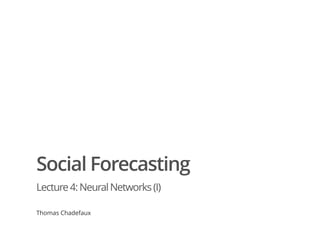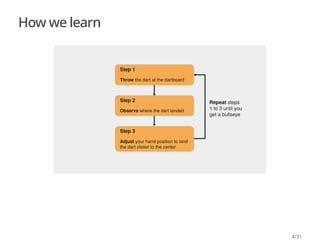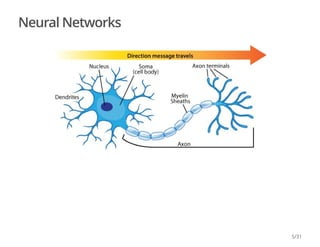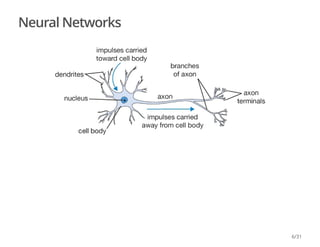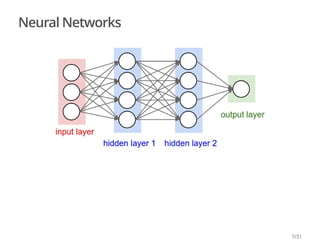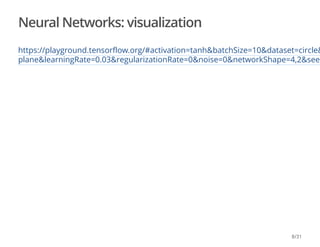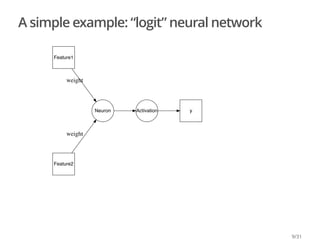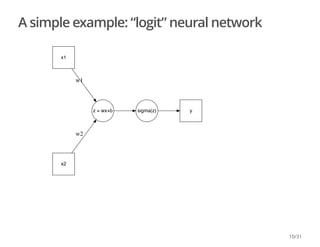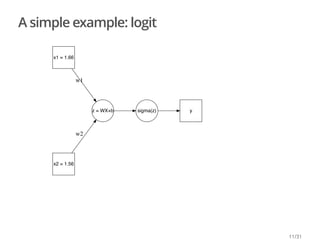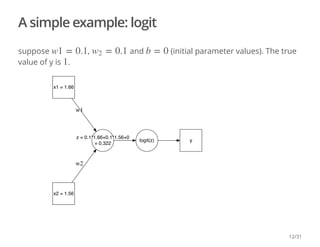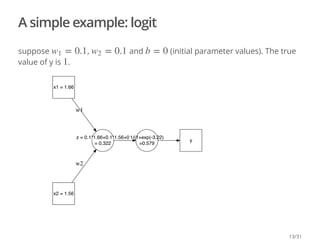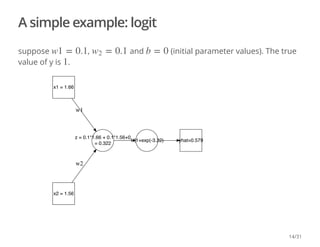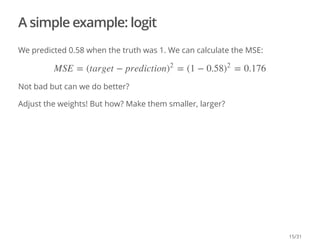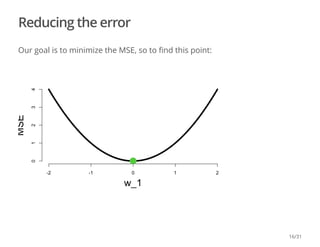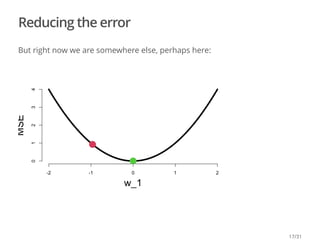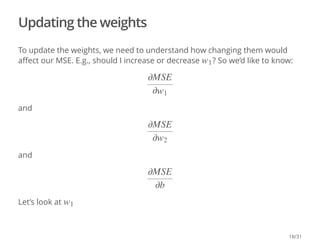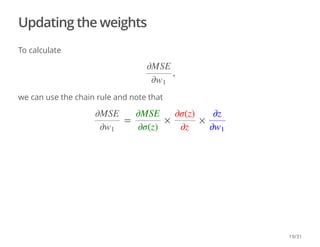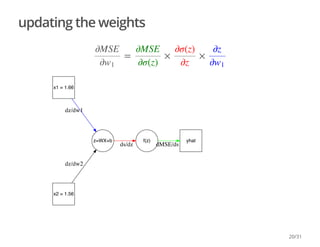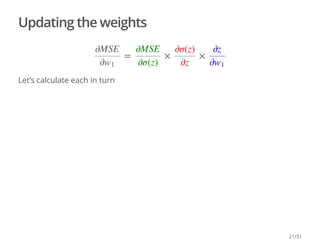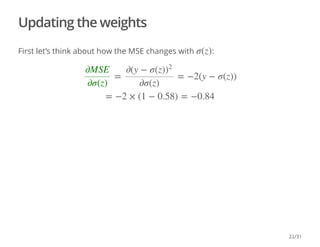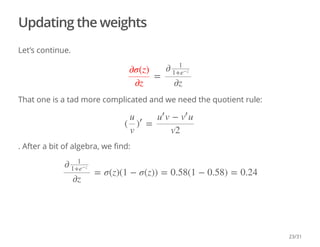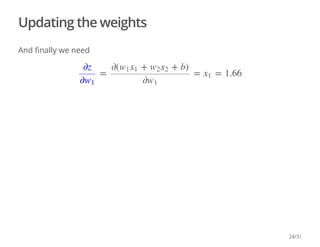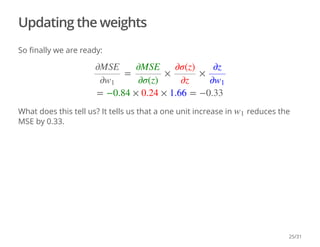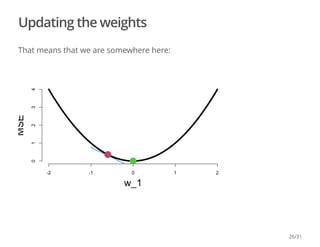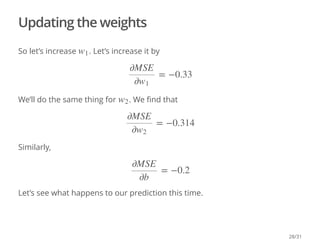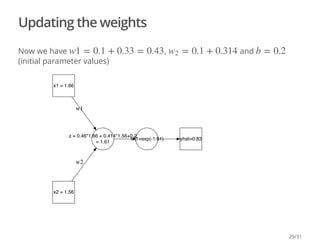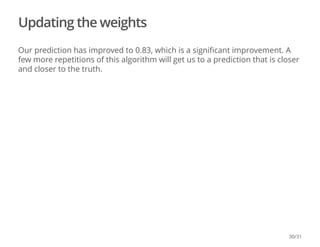1 de 31
Anúncio

### lecture4.pdf

1. Social Forecasting Lecture4:NeuralNetworks(I) Thomas Chadefaux
2. Neural Networks
3. How we learn 3/31
4. How we learn 4/31
5. Neural Networks 5/31
6. Neural Networks 6/31
7. Neural Networks 7/31
8. Neural Networks: visualization https://playground.tensorflow.org/#activation=tanh&batchSize=10&dataset=circle& plane&learningRate=0.03&regularizationRate=0&noise=0&networkShape=4,2&see 8/31
9. A simple example: “logit” neural network Neuron Activation y Feature1 weight Feature2 weight 9/31
10. A simple example: “logit” neural network z = wx+b sigma(z) y x1 w1 x2 w2 10/31
11. A simple example: logit z = WX+b sigma(z) y x1 = 1.66 w1 x2 = 1.56 w2 11/31
12. A simple example: logit suppose , and (initial parameter values). The true value of y is . z = 0.1*1.66+0.1*1.56+0 = 0.322 logit(z) y x1 = 1.66 w1 x2 = 1.56 w2 w1 = 0.1 = 0.1 w2 b = 0 1 12/31
13. A simple example: logit suppose , and (initial parameter values). The true value of y is . z = 0.1*1.66+0.1*1.56+0 = 0.322 1/(1+exp(-3.22) =0.579 y x1 = 1.66 w1 x2 = 1.56 w2 = 0.1 w1 = 0.1 w2 b = 0 1 13/31
14. A simple example: logit suppose , and (initial parameter values). The true value of y is . z = 0.1*1.66 + 0.1*1.56+0 = 0.322 1/(1+exp(-3.22) yhat=0.579 x1 = 1.66 w1 x2 = 1.56 w2 w1 = 0.1 = 0.1 w2 b = 0 1 14/31
15. A simple example: logit We predicted 0.58 when the truth was 1. We can calculate the MSE: Not bad but can we do better? Adjust the weights! But how? Make them smaller, larger? MSE = (target − prediction = (1 − 0.58 = 0.176 ) 2 ) 2 15/31
16. Reducing the error Our goal is to minimize the MSE, so to find this point: 16/31
17. Reducing the error But right now we are somewhere else, perhaps here: 17/31
18. Updating the weights To update the weights, we need to understand how changing them would affect our MSE. E.g., should I increase or decrease ? So we’d like to know: and and Let’s look at w1 ∂MSE ∂w1 ∂MSE ∂w2 ∂MSE ∂b w1 18/31
19. Updating the weights To calculate we can use the chain rule and note that , ∂MSE ∂w1 = × × ∂MSE ∂w1 ∂MSE ∂σ(z) ∂σ(z) ∂z ∂z ∂w1 19/31
20. updating the weights z=WX+b f(z) ds/dz yhat dMSE/ds x1 = 1.66 dz/dw1 x2 = 1.56 dz/dw2 = × × ∂MSE ∂w1 ∂MSE ∂σ(z) ∂σ(z) ∂z ∂z ∂w1 20/31
21. Updating the weights Let’s calculate each in turn = × × ∂MSE ∂w1 ∂MSE ∂σ(z) ∂σ(z) ∂z ∂z ∂w1 21/31
22. Updating the weights First let’s think about how the MSE changes with : σ(z) = = −2(y − σ(z)) ∂MSE ∂σ(z) ∂(y − σ(z)) 2 ∂σ(z) = −2 × (1 − 0.58) = −0.84 22/31
23. Updating the weights Let’s continue. That one is a tad more complicated and we need the quotient rule: . After a bit of algebra, we find: = ∂σ(z) ∂z ∂ 1 1+e −z ∂z ( = u v ) ′ v − u u ′ v ′ v2 = σ(z)(1 − σ(z)) = 0.58(1 − 0.58) = 0.24 ∂ 1 1+e −z ∂z 23/31
24. Updating the weights And finally we need = = = 1.66 ∂z ∂w1 ∂( + + b) w1x1 w2x2 ∂w1 x1 24/31
25. Updating the weights So finally we are ready: What does this tell us? It tells us that a one unit increase in reduces the MSE by 0.33. = × × ∂MSE ∂w1 ∂MSE ∂σ(z) ∂σ(z) ∂z ∂z ∂w1 = −0.84 × 0.24 × 1.66 = −0.33 w1 25/31
26. Updating the weights That means that we are somewhere here: 26/31
27. Updating the weights So we should decrease the weight if we want to reduce our MSE. NB: that’s just gradient descent: to find a minimum, take repeated steps in the opposite direction of the gradient. 27/31
28. Updating the weights So let’s increase . Let’s increase it by We’ll do the same thing for . We find that Similarly, Let’s see what happens to our prediction this time. w1 = −0.33 ∂MSE ∂w1 w2 = −0.314 ∂MSE ∂w2 = −0.2 ∂MSE ∂b 28/31
29. Updating the weights Now we have , and (initial parameter values) z = 0.46*1.66 + 0.414*1.56+0.2 = 1.61 1/(1+exp(-1.61) yhat=0.83 x1 = 1.66 w1 x2 = 1.56 w2 w1 = 0.1 + 0.33 = 0.43 = 0.1 + 0.314 w2 b = 0.2 29/31
30. Updating the weights Our prediction has improved to 0.83, which is a significant improvement. A few more repetitions of this algorithm will get us to a prediction that is closer and closer to the truth. 30/31
31. What next? More neurons, more layers 31/31
Anúncio